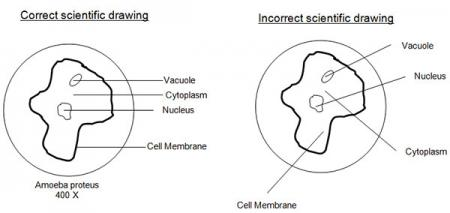# Quantitative Vs. Qualitative Data

Collecting data is an important part of any scientific investigation. There are two types of data. Quantitative data involves numbers, such as "the length of the specimen is 4 centimeters." Qualitative data involves descriptions, characteristics, or features, such as "bubbles formed when the two liquids were combined."

The chart below describes the differences in quantitative and qualitative data.

 Qualitative Date Quantitative Data Data that describes the characteristics such as colors, smells texture, etc. Qualitative → Quality Example: It is cold outside. Data which can be measured such as length, time, temperature, etc. Quantitative → Quantity Example: The temperature outside is 1.1 degree Celsius.

# Accuracy and Precision

Accuracy and precision have very similar definitions—both describe the quality of results in a laboratory experiment. The difference between the definitions, however, is very important. Accuracy describes how close values are to widely accepted or published values. For example, if you measured the acceleration due to gravity, did you get 9.8 m/s2? Precision describes the quality of your measurements themselves. For example, how sure are you of them, and if you measure again, will you get the same result?

Measurements are an important part of doing experiments. Two different and often confused concepts pertaining to measurements are accuracy and precision.

Accuracy is how close to "true" measurements are. Measuring devices or techniques can easily be inaccurate and lead to false measurements, and no matter how accurate a device may be, there is still a tolerance for error. No measurement is perfect. Accuracy must be accounted for in your results.

Precision is how consistent your results are for the same phenomena over several measurements, or how repeatable a device's (like a spring's) performance can be made. Precision as a measure of variation, must be accounted for in your calculations and results.

Watch this video from TedEd  to answer the question, "What's the difference between accuracy and precision?"

Cite Source
Anticole, Matt (2015, April 14). What's the difference between accuracy and precision? [Video file]. Retrieved from https://www.youtube.com/watch?v=hRAFPdDppzs

Now that you have had time to explore the differences between accuracy and precision, take a look at the following diagrams that show the results of four different rounds of a dart game. Drag each tile to the appropriate picture of the dartboard.

Look at the list below of descriptors for accuracy or precision. Decide if the statement is referring to accuracy or precision. Drag the statement into the appropriate column.

# Reading a Metric Ruler

The standard unit for measurement of length in the metric system is the meter. In science class, most measurements are much smaller than a meter. A metric ruler is the standard instrument for measurement in the scientific laboratory. On a metric ruler, each individual line represents a millimeter (mm). The numbers on the ruler represent centimeters (cm). There are 10 millimeters for each centimeter. To read a metric ruler, each individual line represents .10 (1/10) of a centimeter, or 1 millimeter.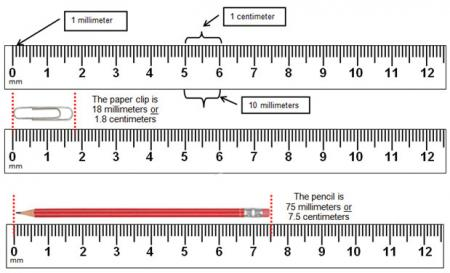Notice when converting from centimeters to millimeters, the decimal point is moved one place to the right. To convert millimeters to centimeters, move the decimal point one place to the left.

Sources: from top to bottom as they appear in this section:
Paper Clip, Knowledge Tree
Pencil, The Vivid Edge

A graduated cylinder is used to measure liquid volume. The unit is the milliliter (mL). To use a graduated cylinder, you must remember the following: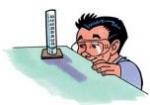• Place the graduated cylinder on a flat surface and view the height of the liquid in the cylinder with your eyes directly level with the liquid. The liquid will tend to curve downward. This curve is called the meniscus. Always read the measurement at the bottom of the meniscus.The graduated cylinder will usually have heavy markings at 10, 20, 30, etc. milliliters. There are smaller markings in between the larger units called graduations. Read the graduated cylinder to the nearest tenth of a milliliter (46.5 mL or 20.0 mL).

• Graduated cylinders come in many different sizes (including 10 mL, 25 mL, 50 mL, 100 mL, 500 mL, and 1000 mL) and can be marked with different scale increments. It is important to determine the scale increment before you begin to measure. To find the scale increment, subtract the values of any two adjacent labeled graduations and divide by the number of intervals between them. This technique works for thermometers, spring scales, and other measuring instruments as well.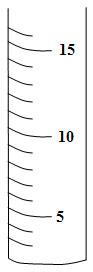For example:
15 – 10 = 5 (subtract the values of two adjacent labeled graduations)

5/5= 1 (divide by the number of intervals between them)

Therefore, each graduation on the cylinder is 1 mL

Sources: as they appear in the section, from top to bottom:
Graduated Cylinder, The Science Network

# Reading a Thermometer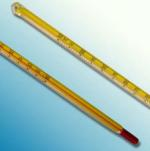A thermometer is a tool or instrument used to measure temperature. Thermometers should be handled with care. They are tubes of glass filled with colored alcohol. At the bottom of the tube is a wider part called the bulb. When the bulb is heated, the liquid in the bulb expands or gets larger, causing the liquid to rise in the tube. When the bulb is cooled, the liquid contracts or gets smaller, causing the liquid to fall in the tube. The bulb at the base of the thermometer should be immersed in the material to be measured. To use a thermometer, you must remember the following:

• In science, temperature is measured in degrees Celsius (°C).
• If you are measuring the temperature of a material that is being heated, make sure the thermometer bulb is not resting on the bottom of the container.
• To read the temperature, your eyes should be level with the liquid in the thermometer.
• Determine the increment scale in the same manner you did the graduated cylinder.

# Reading a Triple Beam Balance

Balances are used to measure mass. The units of mass are grams (g). The instrument you will use most often to measure mass is the triple beam balance. The triple beam balance gets its name from three long, horizontal metal bars called beams connected to a pointer on the right-hand side. The pointer should always be at zero point before obtaining the mass of any object. On each beam is a rider that slides across the beam. Before using a triple beam balance, remember the following:

• Slide all riders back to "0" on the beams before massing any object. Use the adjustment knob to zero out the instrument if necessary.
• Place the object to be massed on the pan. Chemicals should be placed on waxed or filter paper before massing.
• Gently slide the riders along the beams while determining mass. The pointer will swing above and below the zero point while you are adjusting the riders.
• Make sure each rider is in a notch before reading.
• The mass of the object will be the sum of the masses on all beams (measured to a tenth of a gram).
• Always return the riders to zero BEFORE removing the object from the pan.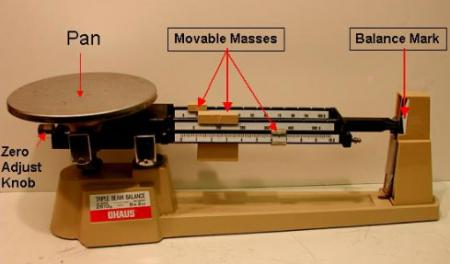To practice using a triple beam balance, go to the link below and click through the following tutorial.

Reading a Triple Beam Balance

Note: Adobe Flash Required. This interactive may not work on all devices and is not compatible with Google Chrome.

# Making Scientific Drawings

Scientific drawings can be made using several methods, depending on a particular laboratory investigation. For a microscopy lab, the drawings are made in circles that represent the viewing field of a microscope. For other labs such as dissection labs, the drawings are representative of the entire organism or parts of the organism. These drawings show the relative size, shape, and location of anatomical structures. Most scientific drawings are labeled.

Use the following guidelines to help make your scientific drawing as clear as possible:

• Use a pencil.
• Title your drawing.
• Be sure that the size of the drawing is proportional to the way it appears in the field of view.
• Use a ruler to draw label lines.
• Do not use arrows for label lines.
• Be sure that label lines point to the center of the structure being labeled.
• Print all labels horizontally.
• Label the right-hand side of the drawing, if possible.
• Do not cross label lines.
• If color is used, be sure that it accurately represent the color of the object.
• Include magnification (if a microscopic drawing) or a scale (if a macroscopic drawing.)